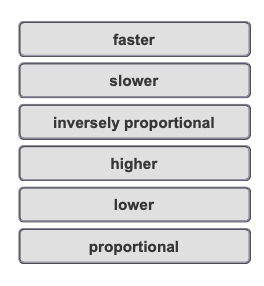# Problem: A glass tube contains an equal number of moles of helium and argon. After five minutes, half of the particles escape through a small hole in the glass.What are the relative amounts of helium and argon in the tube at five minutes?Match the words in the left column to the appropriate blanks in the sentences on the right.The rate of effusion is ______ to the square root of the molar mass. Since the molar mass of helium is _______  than the molar mass of argon, helium will effuse _______ . Therefore, after five minutes when the half of particles escape, the number of moles of helium will be ________ than the number of moles of argon.

###### FREE Expert Solution

The rate of effusion is inversely proportional to the square root of the molar mass.###### Problem Details

A glass tube contains an equal number of moles of helium and argon. After five minutes, half of the particles escape through a small hole in the glass.

What are the relative amounts of helium and argon in the tube at five minutes?

Match the words in the left column to the appropriate blanks in the sentences on the right.The rate of effusion is ______ to the square root of the molar mass. Since the molar mass of helium is _______  than the molar mass of argon, helium will effuse _______ . Therefore, after five minutes when the half of particles escape, the number of moles of helium will be ________ than the number of moles of argon.